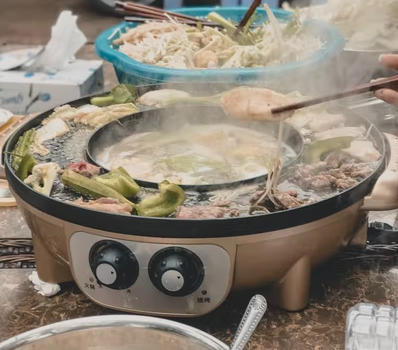## Hot Pot

A 120 V hot pot for cooking is designed to use a 20 ohm resistor. Calculate the power (W) that is consumed by the electric device when in use.Hint
Power absorbed by a resistive element:
$$P=VI=\frac{V^{2}}{R}=I^2R$$$where $$V$$ is voltage, $$I$$ is current, and $$R$$ is resistance. Hint 2 Based on the problem statement’s givens, solve for power using the equation: $$P=\frac{V^{2}}{R}$$$
Power absorbed by a resistive element:
$$P=VI=\frac{V^{2}}{R}=I^2R$$$where $$V$$ is voltage, $$I$$ is current, and $$R$$ is resistance. Solving for Power using the problem statement’s knowns: $$P=\frac{V^{2}}{R}=\frac{120^{2}}{20}$$$
$$P=\frac{14,400}{20}=720\:W$$\$
720 W